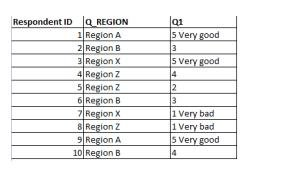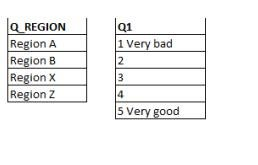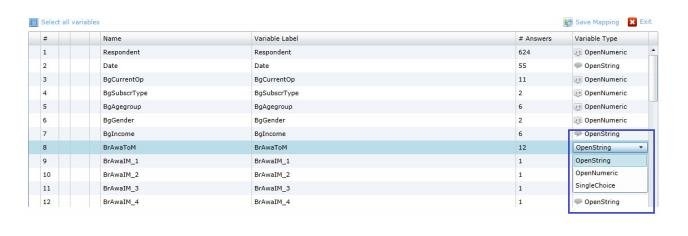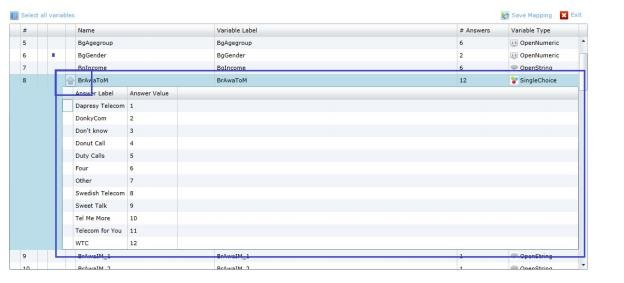If data is imported through the Excel/CSV format this function can be used to create Categorical variables from String or Numeric variables. The recoding is completely automatic and all distinct options in the String/Numeric variable will be recoded to a categorical answer option. This means that the recoding function is great to use in cases where the variables have a fixed list of options. It is not meant to be used where a fixed list is not present (e.g. recoding top of mind brands in a brand study as that needs a more dynamic recoding logic).

Example: In the example below the string questions Q_Region and Q1 is being recoded into a Categorical question. The first image below shows data and the second image shows the answer blocks that will be created during the re coding. The image below shows the imported data.The image below shows the answer block that will be created during the re coding.To recode a variable into a categorical variable the Meta data transformation is used. This means that the recoding must be done the first time the variable is being imported as it is not supported to change variable type afterwards. To recode a string/numeric simply change variable type in the most right column as shown in the image below. When doing that all distinct options in the current data file is shown as answer alternatives as shown in the second image below.

The image below shows where to change variable typeThe image below shows the created answer options, these appears when the type is changed from String/numeric to Single choice. Click the Arrow to view the answer options. In this mode the answer texts can be updated.If a Numeric variable is recoded into a Categorical variable the numeric value will be used as both Answer ID and Answer Text as default. The Answer text can be updated in the Meta data transformation tool before the import and it is highly recommended as all variables with same Answer text will share answer block.

The option to recode string variable to categorical is supported for SPSS, Excel and Triple-S data files imported